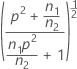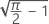# Methods and formulas for Power and Sample Size for 2 Variances

Select the method or formula of your choice.

## Calculating power with Levene's method

The power function for a variance test is Q(ρ) = P(Reject H0 | ρ).

### Notation

TermDescription
ΦCDF of the standard normal distribution
tα d upper percentile of the t distribution with d = n1 + n2 – 2 degrees of freedom
θcρ σ 1 / σ 2
n 1 size of the first sample
n 2 size of the second sample

## Calculating power with the F-test method

### Formula

The power function for a variance test is Q(ρ) = P(Reject H0 | ρ).

### Notation

TermDescription
F k 1, k 2 distribution function of the F distribution with k1 and k2 degrees of freedom
v k 1, k 2, A inverse CDF evaluated at A for an F distribution with k 1 and k 2 degrees of freedom
k 1 n – 1
k2 n – 1
α significance level
ρ σ 1 / σ 2

## Calculating power using Bonett's method

###### Note

To calculate power for the Bonett method, use the session command POWER with the subcommands TWOVARIANCE and BONETT.

The power function for a variance test is Q(ρ) = P (Reject H0 | ρ). For Bonett's test, the calculations for the power function are based on the statistic Z. For large samples, Z is approximately distributed as the standard normal distribution. Z is given by:
where se is the standard error, which is given by:

### Notation

TermDescription
Sithe standard deviation of sample i
ρthe ratio of the population standard deviations ( s1 / s2)
sethe standard error
γthe true common kurtosis of the parent populations (γ is not the excess kurtosis)
nthe sample size (For power calculations, n is assumed to be the same for both samples)
Φthe cumulative distribution function for the standard normal distribution
αthe significance level for the test
zithe upper i percentile point for the standard normal distribution

## Calculating sample size and ratio

If you provide values for power and sample size, Minitab calculates the value of the ratio. If you provide values for power and ratio, Minitab calculates the value of the sample size.

For these two cases, Minitab uses an iterative algorithm with the power equation. At each iteration, Minitab evaluates the power for a trial sample size or trial ratio, and stops when it reaches the values you request.

### Target power and actual power

When Minitab calculates sample size, it may find that no integer value of sample size yields your target power. In such cases, Minitab displays the target value for power alongside the actual power, which is a value corresponding to an integer sample size, and which is nearest to, yet greater than, the target value.

By using this site you agree to the use of cookies for analytics and personalized content.  Read our policy